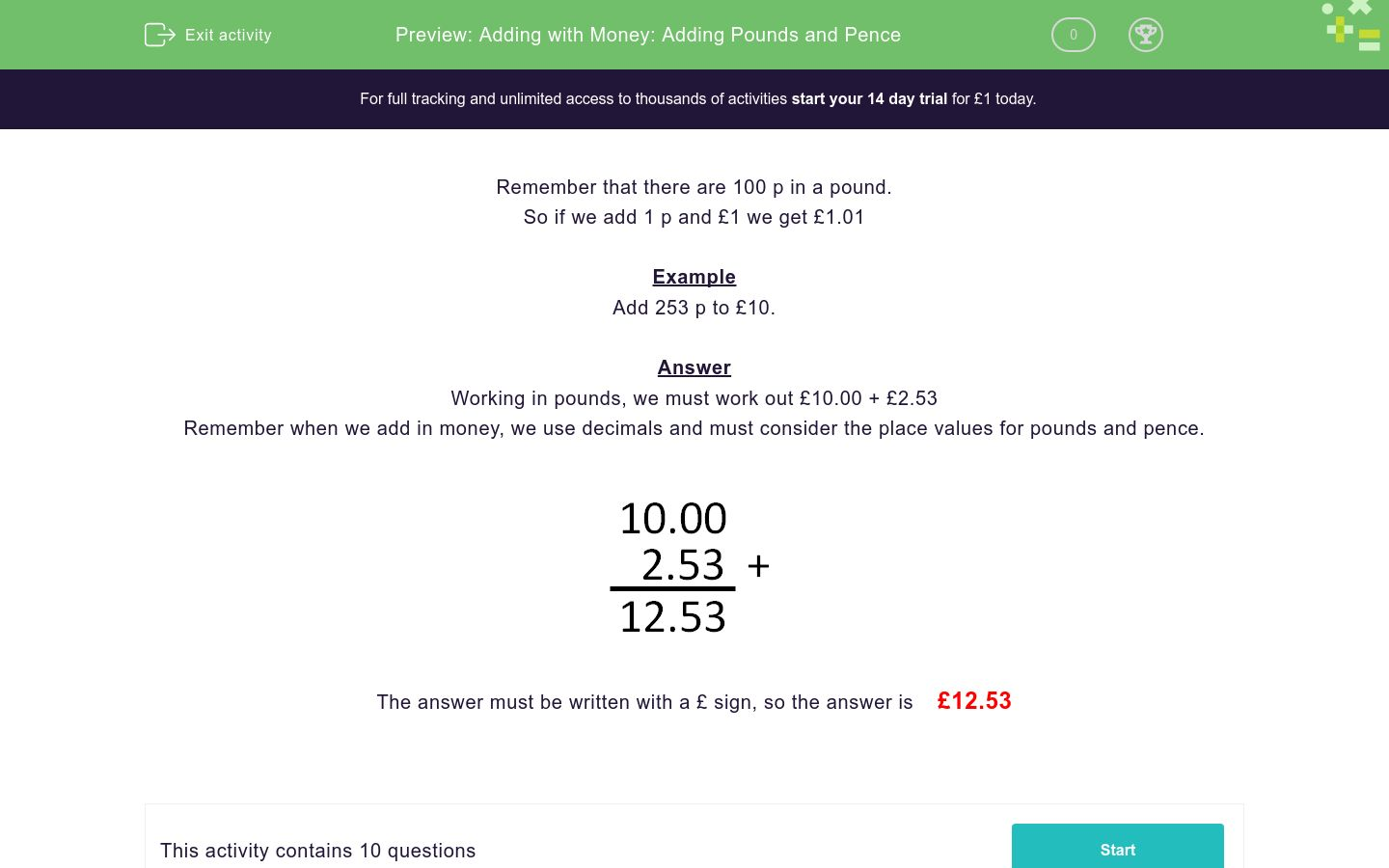### Affordable pricing from £10/month

In this worksheet, students add the given amounts of money and write their answers in pounds.Key stage:  KS 2

Curriculum topic:   Measurement

Curriculum subtopic:   Solve Four Operations Problems

Difficulty level:### QUESTION 1 of 10

Remember that there are 100 p in a pound.

So if we add 1 p and £1 we get £1.01

Example

Working in pounds, we must work out £10.00 + £2.53

Remember when we add in money, we use decimals and must consider the place values for pounds and pence.The answer must be written with a £ sign, so the answer is    £12.53

Work out:

£2 + 15p

Work out:

£12 + 15p

Work out:

£10 + 45p

Work out:

£10 + 145p

Work out:

£10 + 347p

Work out:

£100 + 20p

Work out:

£100 + 220p

Work out:

£100 + 298p

Work out:

£100 + 1205p

Work out:

£100 + 1234p

• Question 1

Work out:

£2 + 15p

£2.15
EDDIE SAYS
Add £2.00 + £0.15 1. Add the hundredths, 0 + 5 = 5. 2. Add the tenths, 0 + 1 = 1. 3. Add the ones 2 + 0 = 2. Answer: £2.15
• Question 2

Work out:

£12 + 15p

£12.15
EDDIE SAYS
Add £12.00 + £0.15 1. Add the hundredths, 0 + 5 = 5. 2. Add the tenths, 0 + 1 = 1. 3. Add the ones 2 + 0 = 2 and add the tens, 1 + 0 = 1. Answer: £12.15
• Question 3

Work out:

£10 + 45p

£10.45
EDDIE SAYS
Add £10.00 + £0.45 1. Add the hundredths, 0 + 5 = 5. 2. Add the tenths, 0 + 4 = 4. 3. Add the ones 0 + 0 = 0 and add the tens, 1 + 0 = 1. Answer: £10.45
• Question 4

Work out:

£10 + 145p

£11.45
EDDIE SAYS
Add £10.00 + £1.45 1. Add the hundredths, 0 + 5 = 5. 2. Add the tenths, 0 + 4 = 4. 3. Add the ones 0 + 1 = 1 and add the tens, 1 + 0 = 1. Answer: £11.45
• Question 5

Work out:

£10 + 347p

£13.47
EDDIE SAYS
Add £10.00 + £3.47 1. Add the hundredths, 0 + 7 = 7. 2. Add the tenths, 0 + 4 = 4. 3. Add the ones 0 + 3 = 3 and add the tens, 1 + 0 = 1. Answer: £13.47
• Question 6

Work out:

£100 + 20p

£100.20
EDDIE SAYS
Add £100.00 + £0.20 1. Add the hundredths, 0 + 0 = 0. 2. Add the tenths, 0 + 2 = 2. 3. Add the ones 0 + 0 = 0, add the tens, 0 + 0 = 0 and add the hundreds 1 + 0 = 1. Answer: £100.20
• Question 7

Work out:

£100 + 220p

£102.20
EDDIE SAYS
Add £100.00 + £2.20 1. Add the hundredths, 0 + 0 = 0. 2. Add the tenths, 0 + 2 = 2. 3. Add the ones 0 + 2 = 2, add the tens, 0 + 0 = 0 and add the hundreds 1 + 0 = 1. Answer: £102.20
• Question 8

Work out:

£100 + 298p

£102.98
EDDIE SAYS
Add £100.00 + £2.98 1. Add the hundredths, 0 + 8 = 8. 2. Add the tenths, 0 + 9 = 9. 3. Add the ones 0 + 2 = 2, add the tens, 0 + 0 = 0 and add the hundreds 1 + 0 = 1. Answer: £102.98
• Question 9

Work out:

£100 + 1205p

£112.05
EDDIE SAYS
Add £100.00 + £12.05 1. Add the hundredths, 0 + 5 = 5. 2. Add the tenths, 0 + 0 = 0. 3. Add the ones 0 + 2 = 2, add the tens, 0 + 1 = 1 and add the hundreds 1 + 0 = 1. Answer: £112.05
• Question 10

Work out:

£100 + 1234p

£112.34
EDDIE SAYS
Add £100.00 + £12.34 1. Add the hundredths, 0 + 4 = 4. 2. Add the tenths, 0 + 3 = 3. 3. Add the ones 0 + 2 = 2, add the tens, 0 + 1 = 1 and add the hundreds 1 + 0 = 1. Answer: £112.34
---- OR ----

Sign up for a £1 trial so you can track and measure your child's progress on this activity.

### What is EdPlace?

We're your National Curriculum aligned online education content provider helping each child succeed in English, maths and science from year 1 to GCSE. With an EdPlace account you’ll be able to track and measure progress, helping each child achieve their best. We build confidence and attainment by personalising each child’s learning at a level that suits them.

Get started